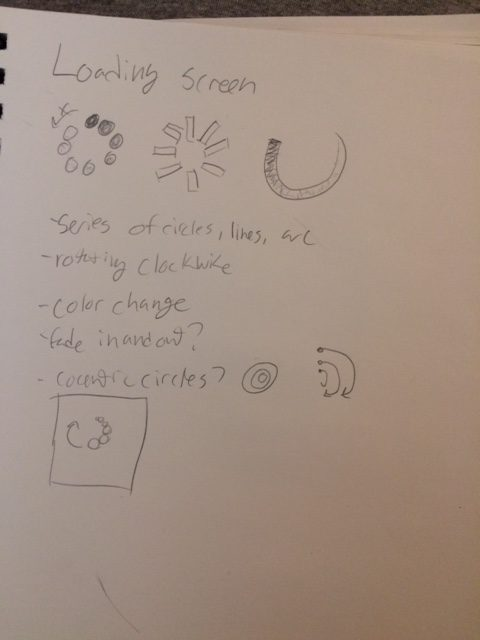# ookey-AnimatedLoop```// Template taken from Prof. Golan Levin // These can then be combined into an animated gif. // Known to work with Processing 3.3.6 // Prof. Golan Levin, January 2018   //=================================================== // Global variables. String myNickname = "ookey"; int nFramesInLoop = 100; int nElapsedFrames; boolean bRecording;   //=================================================== void setup() { size (500, 500); bRecording = false; nElapsedFrames = 0; } //=================================================== void keyPressed() { if ((key == 'f') || (key == 'F')) { bRecording = true; nElapsedFrames = 0; } }   //=================================================== void draw() {   // Compute a percentage (0...1) representing where we are in the loop. float percentCompleteFraction = 0; if (bRecording) { percentCompleteFraction = (float) nElapsedFrames / (float)nFramesInLoop; } else { percentCompleteFraction = (float) (frameCount % nFramesInLoop) / (float)nFramesInLoop; }   // Render the design, based on that percentage. renderMyDesign (percentCompleteFraction);   // If we're recording the output, save the frame to a file. if (bRecording) { saveFrame("frames/" + myNickname + "_frame_" + nf(nElapsedFrames, 4) + ".png"); nElapsedFrames++; if (nElapsedFrames &gt;= nFramesInLoop) { bRecording = false; } } }   //=================================================== void renderMyDesign (float percent) {   //---------------------- background (#4571DA); smooth(); stroke (0, 0, 0); strokeWeight (2);   //--------------------- float cx = width/2; float cy = height/2; float radius; float angle; float px; float py;     for (int i = 0; i &lt; 20; i++) { radius = 100; angle = function_PennerEaseInOutElastic(percent) * TWO_PI + (PI/40 * i); px = cx + radius*cos(angle); py = cy + radius*sin(angle); fill(color(255, 255, 255, 255 * map(cos(angle), 0., 1., 1., 0.05) * (.7 + function_PennerEaseInOutBack(sin(PI * percent))))); noStroke(); ellipse (px, py, 20 + i, 20 + i); }   }   // Taken from https://github.com/golanlevin/Pattern_Master float function_PennerEaseInOutElastic (float t) { // functionName = "Penner's EaseInOut Elastic";   if (t==0) return 0; if ((t/=0.5)==2) return 1; float p=(.3f*1.5f); float a=1; float s=p/4;   if (t &lt; 1) { float postFix = pow(2, 10*(t-=1)); // postIncrement is evil return -0.5f*(postFix* sin( (t-s)*(2*PI)/p )); } float postFix = pow(2, -10*(t-=1)); // postIncrement is evil return postFix * sin( (t-s)*(2*PI)/p )*.5f + 1; }   float function_PennerEaseInOutBack (float x) { // functionName = "Penner's EaseInOut Back";   float s = 1.70158 * 1.525; x /= 0.5;   float y = 0; if (x &lt; 1) { y = 1.0/2.0* (x*x*((s+1.0)*x - s)); } else { x -= 2.0; y = 1.0/2.0* (x*x*((s+1.0)*x + s) + 2.0); } return y; }```